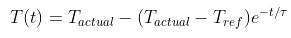## Temperature Probe

Consider a temperature probe, with the below controlling equation and a time constant of 5 seconds, is stuck inside a cup of liquid. What is the minimum time required before the probe indicates a temperature within ±2°C of the actual liquid temperature if the actual liquid and reference temperatures are 150°C and 50°C respectively?Hint
Minimum time required means:
$$T(t)=T_{actual}-2^{\circ}C=150^{\circ}C-2^{\circ}C=148^{\circ}C$$$Hint 2 Solve for t: $$148^{\circ}C=150^{\circ}C-(150^{\circ}C-50^{\circ}C)e^{-t/5s}$$$
Minimum time required means:
$$T(t)=T_{actual}-2^{\circ}C=150^{\circ}C-2^{\circ}C=148^{\circ}C$$$Thus, we can solve for the minimum time (t): $$148^{\circ}C=150^{\circ}C-(150^{\circ}C-50^{\circ}C)e^{-t/5s}$$$
$$(100^{\circ}C)e^{-t/5s}=150^{\circ}C-148^{\circ}C$$$$$e^{-t/5s}=\frac{2^{\circ}C}{100^{\circ}C}=0.02$$$
$$ln[e^{-t/5s}]=ln[0.02]$$$$$\frac{-t}{5s}=-3.912$$$
$$t=3.912(5s)=19.56\:sec$$\$
19.56 s